# Fourier Transform

## 1. The Fourier Transform

The Fourier transformof an integrable functionwith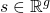is given by

(1)Under suitable conditions, the inverse transform fromto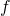withis then given by

(2)The Fourier transform has some nice properties. Assume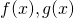andare integrable functions:

1. Linearity: For, if, then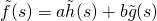.
2. Translation: For, if, then.
3. Modulation: For, if, then.
4. Scaling: For, if, then.

Here we use the notation is used.

An important feature of the Fourier Transform is convolution. Suppose two given functionsand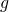and let the convolution be defined as, thenand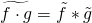.

## 2. Discrete Fourier Transform

The discrete Fourier Transform (DFT) is based on points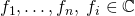and given by

(3)and the inverse by

(4)The DFT can be interpet as a discrete version of 1. To see this, letbe some grid. Then a discrete, approximate version of (1) is given by

(5)Ifare g-dimensional equidistantly spaced then (5) will be the Riemann sum while for g-dimensional i.i.d. random observations one may interpret (5)  as an monte carlo intergral.

Looking at (1) with integration by substitution with. Assume now observations at an equidistant univariate grid on,, and defineand, then (5) corresponds to (4).

### 1 thought on “Fourier Transform”

This site uses Akismet to reduce spam. Learn how your comment data is processed.